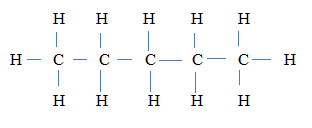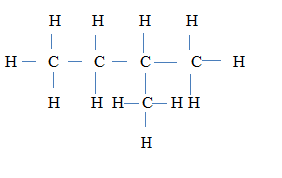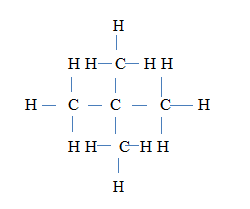### Question 3

1. Define the term structural formula.

2. Draw the structural isomers of C5H12.

3. Give the IUPAC name of each of the isomers drawn in 3(a)(i).

[8 marks]

1. State two of the observations made by Lord Rutherford in his α-particle scattering experiment.

2. Give the inference he made for each of the observations stated in 3(b)(i).

[8 marks]

1. State two of the laws of chemical combination.

2. State the factors that determine whether a given ionic solid would dissolve in water.

3. CO2 and SIO2 are both covalent compounds. Explain briefly why CO2 is a gas whereas SIO2 is a solid under ordinary conditions.

[8 marks]

1. Consider the pH values of the three equimolar solutions, X, Y and Z.

2.  Solution pH Value X 5 Y 2 Z 3

1. acidic.

2. basic.

1. Arrange the solutions in decreasing order of dissociation.

2. Give a reason for the answer stated in 3(d)(ii).

[8 marks]

### Observation

Few candidates responded to this question and their performance in the question was below average.

In part (a), majority of the candidates could not define the term structural formula. Also, they could not draw the structural isomers of pentane correctly.

In part (b), majority of the candidates could not state the observations made by Lord Rutherford in his α-particle scattering experiment.

In part (c), majority of the candidates were able to state the laws of chemical combination. However, many of them could not state the factors that determine solubility of an ionic solid in water.

In part (d), majority of the candidates displayed good knowledge of pH.

1. A ( graphic) representation of a molecule of a compound showing how the atoms are linked together / The arrangement of atoms within the molecule of a substance.

2.3.4.5. pentane
• 2 – methylbutane

• 2, 2 – dimethylpropane

1. i most of the α – particles went straight through the foil
• a few α – particles were deflected through small / fairly large angles

• very few α – particles were repelled back towards the source

2. ii the atoms making up the foil contained a lot of empty spaces
• the positive part of the atom was concentrated in a very small region of
space (the nucleus)

• the nucleus must be very heavy containing almost the entire mass of
the atom

Law of Conservation of matter

• During the course of a chemical reaction, matter is neither created nor destroyed.

Law of Definite proportions

• All pure samples of the same chemical compound contain the same elements combined in the same proportion by mass.

• Law of Multiple proportions

• When two elements A and B combine to form more than one different compounds, the different masses of B that combine separately

with a fixed mass of A are in a simple ratio.

• lattice energy

• hydration energy

• temperature

1. forces between the units / molecules in CO2 are weak van der Waal’s forces whereas forces between the units / atoms in SiO2 are (strong) covalent bonds
1. Solution X

2. Solution Y

1. Solution Y > Solution Z > Solution X / Y, Z, X

2. The lower the pH the stronger the acid hence, the greater the extent of dissociation.